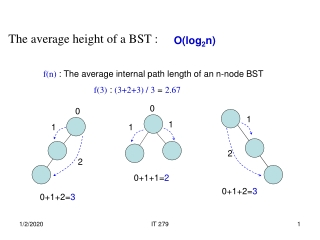DownloadDownload PresentationO(log 2 n)

# O(log 2 n)

Download Presentation## O(log 2 n)

- - - - - - - - - - - - - - - - - - - - - - - - - - - E N D - - - - - - - - - - - - - - - - - - - - - - - - - - -
##### Presentation Transcript

1. The average height of a BST : O(log2n) f(n) : The average internal path length of an n-node BST f(3) : (3+2+3) / 3 = 2.67 0 0 1 1 1 1 2 2 0+1+1=2 0+1+2=3 0+1+2=3 IT 279

2. Adel’son-Vels’kii and Landis, Soviet Mathematic Doklady, 3:1259-1263, 1962 C. Crane, D. Knuth, et al in 1970’s AVL Tree A BST in which the height difference between the two children of any node is always less than 2. +1 +1 -1 j k j+1 k+1 h h+1 IT 279

3. AVL Tree -- an example of Dynamic Tree We dynamicallymaintain the properties of AVL-tree when we insert (remove) a node by four different operations (rotations) Dynamic Tree Static Tree Huffman Code -- an example of Static Tree We staticallyanalyze the code and build up an optimal tree for retrieving the code words. IT 279

4. performed at the bad node where the difference between the heights of its two children is bigger than 1. Four operations : (rotations) If a node is bad caused by: then perform • Right-child’s Right-child • Left-child’s Left-child • Right-child’s Left-child • Left-Child’s Right-child • RR rotation • LL rotation • RL rotation • LR rotation IT 279

5. No Rotation is Needed +1 0 -1 0 IT 279

6. RR Rotations: +2 +1 IT 279

7. RR Rotation: +2 R +0 R +0 +1 IT 279

8. LL Rotation: L -2 +0 L -1 +0 IT 279

9. Rotations: RL +1 +2 0 h+1 +1 -1 0 0 h+1 +0 -1 h h IT 279

10. Rotations: RL RL Rotation: R 0 +2 L h+1 -1 0 +1 h+1 -1 h h h+1 h+1 h h IT 279

11. Rotations: LR LR Rotation: L -2 0 1 R h+1 0 +1 h+1 -1 -1 h h h+1 h+1 h h IT 279

12. Rotate this sub-tree first +2 R Could be RR or RL, depending on what happens in the blue sub-tree. R +2 Afterwards, examine the red node again to see is another rotation is needed. IT 279

13. Rotations: LL +2 +1 +1 h+1 -1 -2 -1 h -2 -1 0 h-1 h -1 0 h-1 h-1 IT 279

14. Example: 10 20 30 10 RR Rotation: 20 20 10 30 30 IT 279

15. Example: 40 50 20 RR Rotation: 20 10 30 10 40 40 50 30 50 IT 279

16. Example: 35 20 R 30 RL Rotation: 10 40 40 L 20 30 50 10 50 35 35 IT 279

17. Possible complications Re-assign the links +2 h+1 -1 h+1 -1 h h Tracking the heights and balance-factors IT 279

18. RR rotation in C++ a=t +2 R b template<typename T> // RR rotation on t; TreeNode<T> * AVLTree<T>::RR(TreeNode<T> * t) { TreeNode<T> * a = t, * b = t->right; a->right = b->left; b->left = a; a->height -= 2; return b; } h R +1 h h height is an extra member variable in the TreeNode. IT 279

19. RL rotation in C++ a=t template<typename T> // RL rotation on t; TreeNode<T> * AVLTree<T>::RL(TreeNode<T> * t) { TreeNode<T> *a, *b, *c; a = t; b = t->right; c = t->right->left; a->right = c->left; b->left = c->right; c->left = a; c->right = b; c->height++; b->height--; a->height-=2; return c; } b R +2 L c h+1 -1 h+1 -1 h h IT 279

20. AVL h: Average Heights n Random Keys IT 279